maths > calculus-limits

Understanding limits with the graph of the function

what you'll learn...

Understanding Limits with Graph of the function

»  Values of Function at x=a$x = a$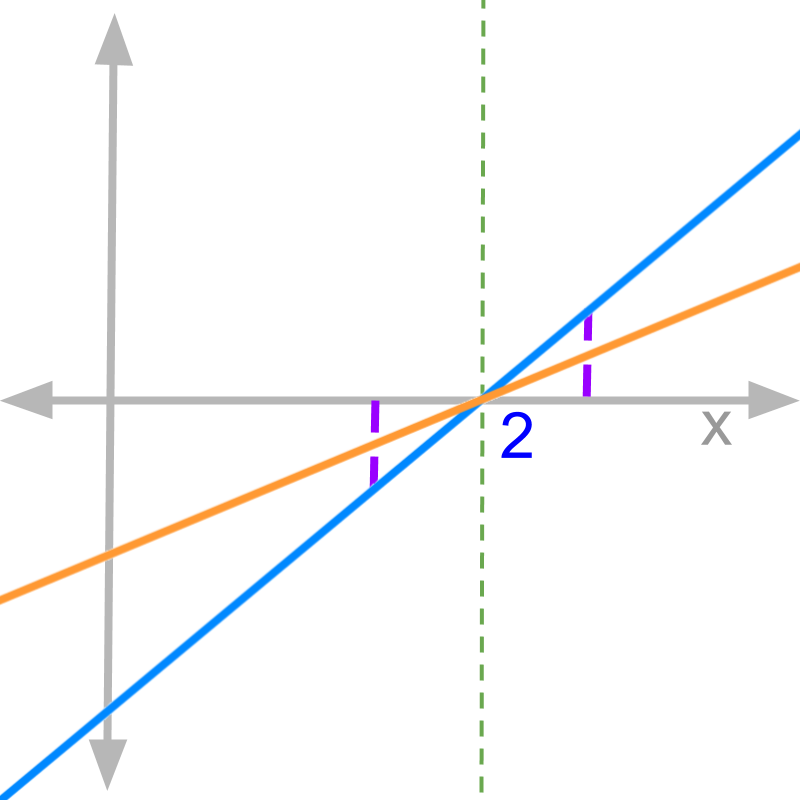→  Evaluated at input f(x)x=a$f \left(x\right) {|}_{x = a}$ or f(a)$f \left(a\right)$

→  Left-hand-limit limxaf(x)${\lim}_{x \to a -} f \left(x\right)$

→  Right-hand-limit limxa+f(x)${\lim}_{x \to a +} f \left(x\right)$

In the previous pages, limit is defined in algebraic form.

In this topic, the function is considered as a graph in a 2D coordinate plane and the meaning of limit is explained.

example

The value of f(x)=x21x1$f \left(x\right) = \frac{\textcolor{\mathrm{de} e p s k y b l u e}{{x}^{2} - 1}}{\textcolor{c \mathmr{and} a l}{x - 1}}$ when $x = 1$ is $\frac{0}{0}$.

On substituting $x = 1$, we get $f \left(1\right) = \frac{0}{0}$.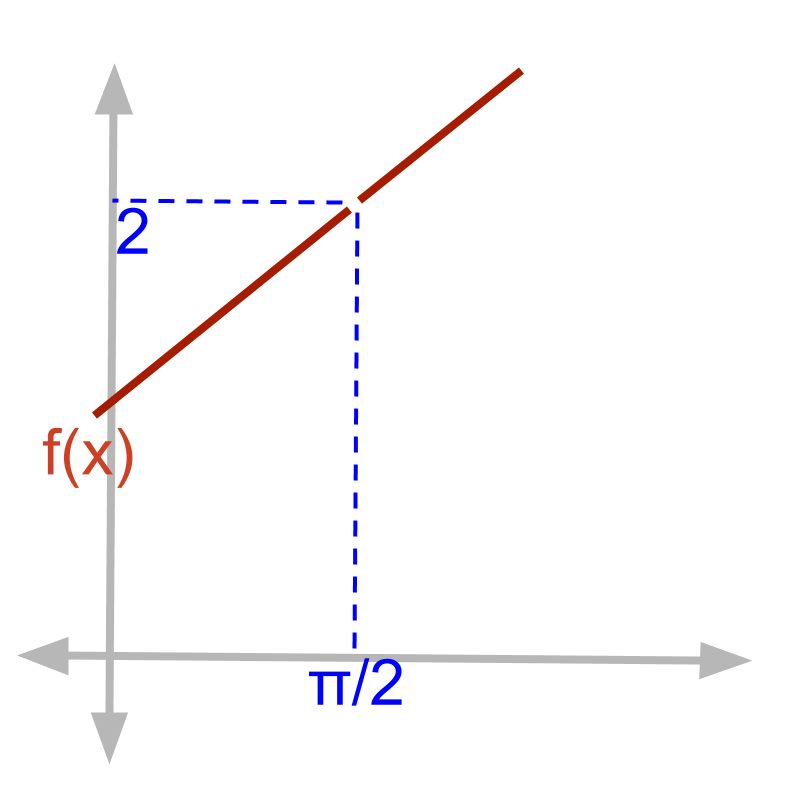The plot of $f \left(x\right) = \frac{\textcolor{\mathrm{de} e p s k y b l u e}{{x}^{2} - 1}}{\textcolor{c \mathmr{and} a l}{x - 1}}$ is shown.

At $x = 1$, the graph breaks and the function does not evaluate to a real number.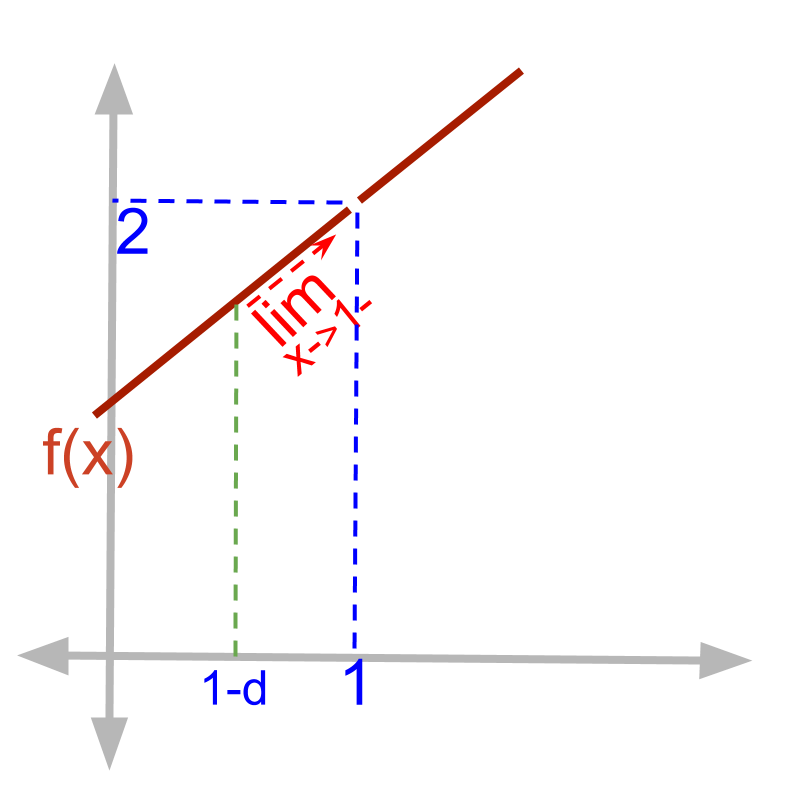Left-hand-limit of $f \left(x\right) = \frac{\textcolor{\mathrm{de} e p s k y b l u e}{{x}^{2} - 1}}{\textcolor{c \mathmr{and} a l}{x - 1}}$ is shown.

At $x = 1 - \delta$, dotted vertical line is shown.

Applying limit is moving the vertical line towards $x = 1$ and making $\delta \cong 0$. This is shown as ${\lim}_{x \to 1 -}$ in the figure.

${\lim}_{x \to 1 -} f \left(x\right)$
$\quad \quad = \frac{\textcolor{\mathrm{de} e p s k y b l u e}{{\left(1 - \delta\right)}^{2} - 1}}{\textcolor{c \mathmr{and} a l}{\left(1 - \delta\right) - 1}}$
$\quad \quad = \frac{\textcolor{\mathrm{de} e p s k y b l u e}{1 - 2 \delta + {\delta}^{2} - 1}}{\textcolor{c \mathmr{and} a l}{1 - \delta - 1}}$
$\quad \quad = \frac{\textcolor{\mathrm{de} e p s k y b l u e}{- \delta \left(2 - \delta\right)}}{\textcolor{c \mathmr{and} a l}{- \delta}}$
$\quad \quad = 2 - \delta$
$\quad \quad = 2$ (substituting $\delta = 0$)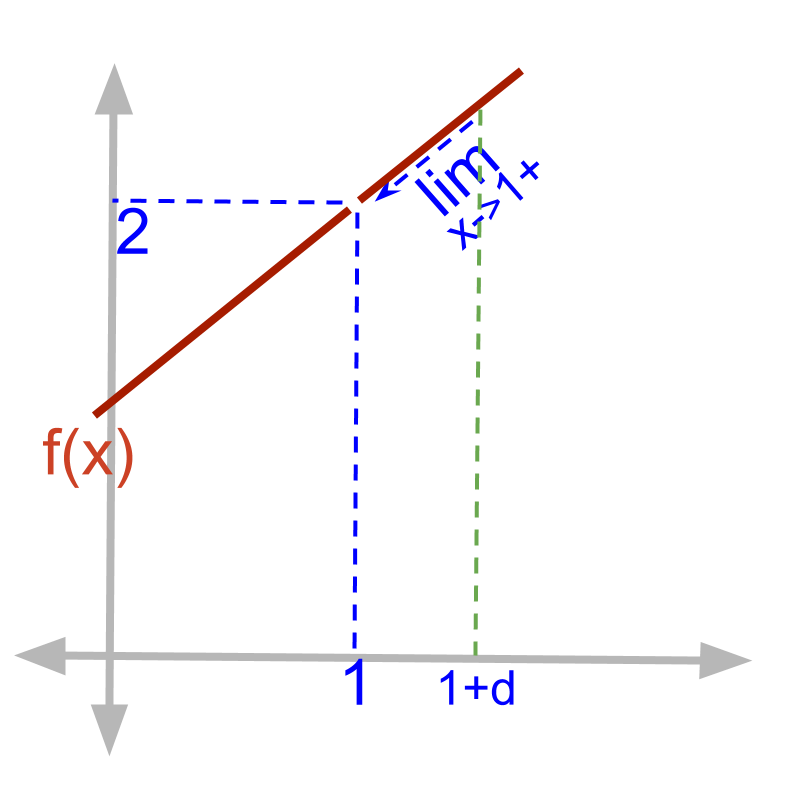Right-hand-limit of $f \left(x\right) = \frac{\textcolor{\mathrm{de} e p s k y b l u e}{{x}^{2} - 1}}{\textcolor{c \mathmr{and} a l}{x - 1}}$ is shown.

At $x = 1 + \delta$, dotted vertical line is shown.

Applying limit is moving the vertical line towards $x = 1$ and making $\delta \cong 0$. This is shown as ${\lim}_{x \to 1 +}$ in the figure.

${\lim}_{x \to 1 +} f \left(x\right)$
$\quad \quad = \frac{\textcolor{\mathrm{de} e p s k y b l u e}{{\left(1 + \delta\right)}^{2} - 1}}{\textcolor{c \mathmr{and} a l}{\left(1 + \delta\right) - 1}}$
$\quad \quad = \frac{\textcolor{\mathrm{de} e p s k y b l u e}{1 + 2 \delta + {\delta}^{2} - 1}}{\textcolor{c \mathmr{and} a l}{1 + \delta - 1}}$
$\quad \quad = \frac{\textcolor{\mathrm{de} e p s k y b l u e}{\delta \left(2 + \delta\right)}}{\textcolor{c \mathmr{and} a l}{\delta}}$
$\quad \quad = 2 + \delta$
$\quad \quad = 2$ (substituting $\delta = 0$)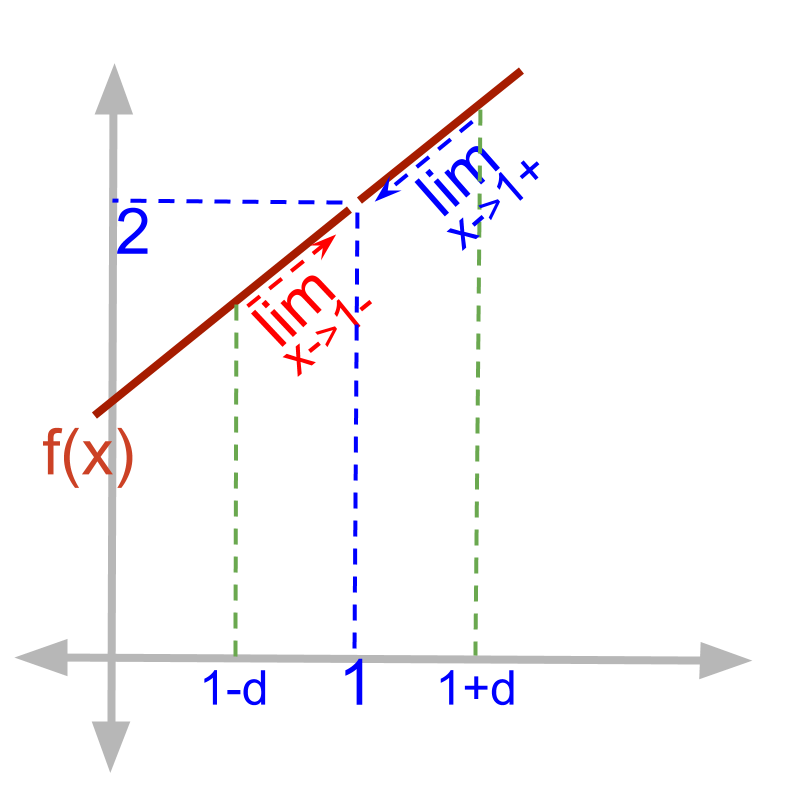Both the limits of $f \left(x\right) = \frac{\textcolor{\mathrm{de} e p s k y b l u e}{{x}^{2} - 1}}{\textcolor{c \mathmr{and} a l}{x - 1}}$ is shown.

The right-hand-limit and left-hand-limits converge to $2$.

Limit of a function at $x = a$ is understood as the value of function at $x = a$, left side of that : $x = a - \delta$, and right side of that : $x = a + \delta$

summary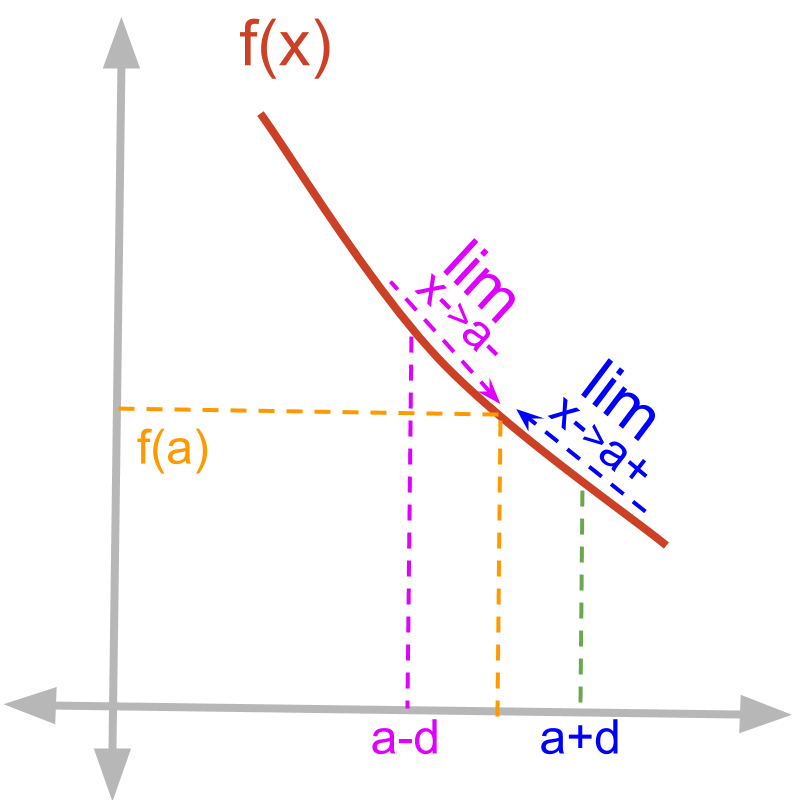Limits of a function at $x = a$ are illustrated in the figure.

•  Evaluated at input $f \left(x\right) {|}_{x = a}$ or $f \left(a\right)$
•  Left-hand-limit ${\lim}_{x \to a -} f \left(x\right)$
•  Right-hand-limit ${\lim}_{x \to a +} f \left(x\right)$

Outline